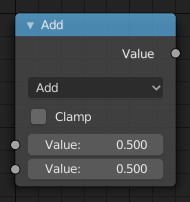# 运算节点¶## 输入选项¶

The inputs of the node are dynamic. Some inputs are only available in certain operations. For instance, the Addend input is only available in the Multiply Add operator.

Base

Input Base.

Exponent

Input Exponent.

Epsilon

Input Epsilon.

Distance

Input Distance.

Min

Input Minimum.

Max

Input Maximum.

Increment

Input Increment.

Scale

Input Scale.

Degrees

Input Degrees.

## 属性¶

The mathematical operator to be applied to the input values:

Functions

The sum of the two values.

Subtract

The difference between the two values.

Multiply

The product of the two values.

Divide

The division of the first value by the second value.

The sum of the product of the two values with Addend.

Power

The Base raised to the power of Exponent.

Logarithm

The log of the value with a Base as its base.

Square Root

The square root of the value.

Inverse Square Root

One divided by the square root of the value.

Absolute

The input value is read with without regard to its sign. This turns negative values into positive values.

Exponent

Raises Euler's number to the power of the value.

Comparison
Minimum

Outputs the smallest of the input values.

Maximum

Outputs the largest of two input values.

Less Than

Outputs 1.0 if the first value is smaller than the second value. Otherwise the output is 0.0.

Greater Than

Outputs 1.0 if the first value is larger than the second value. Otherwise the output is 0.0.

Sign

Extracts the sign of the input value. All positive numbers will output 1.0. All negative numbers will output -1.0. And 0.0 will output 0.0.

Compare

Outputs 1.0 if the difference between the two input values is less than or equal to Epsilon.

Smooth Minimum
Smooth Maximum
Rounding
Round

Round the input value to the nearest integer.

Floor

Rounds the input value down to the nearest integer.

Ceil

Rounds the input value up to the nearest integer.

Truncate

Outputs the integer part of the value.

Fraction
Modulo

Outputs the remainder once the first value is divided by the second value.

Wrap

Outputs a value between Min and Max based on the absolute difference between the input value and the nearest integer multiple of Max less than the value.

Snap

Round the input value to down to the nearest integer multiple of Increment.

Ping-pong

The output value is moved between 0.0 and the Scale based on the input value.

Trigonometric
Sine

The Sine of the input value.

Cosine

The Cosine of the input value.

Tangent

The Tangent of the input value.

Arcsine

The Arcsine of the input value.

Arccosine

The Arccosine of the input value.

Arctangent

The Arctangent of the input value.

Arctan2

Outputs the Inverse Tangent of the first value divided by the second value measured in radians.

Hyperbolic Sine

The Hyperbolic Sine of the input value.

Hyperbolic Cosine

The Hyperbolic Cosine of the input value.

Hyperbolic Tangent

The Hyperbolic Tangent of the input value.

Conversion

Converts the input from degrees to radians.

To Degrees

Converts the input from radians to degrees.

Limits the output to the range (0.0 to 1.0). See Clamp.